# Python Classes and Objects

Classes, an object-oriented feature of Python, provide a way to create programs in a more organized way by putting data members and methods in one place. Class also aids in the incorporation of functionality into objects.

## Creating a class in Python

To create a class in Python, we need to use the "class" keyword, just like creating a function using the "def" keyword. Here is the syntax to create a class in Python:

```class class_name:
statement(s)```

A class contains attributes and behaviors. Here attributes refer to variables, whereas behavior refers to methods (functions).

For example, to create a class named MYCLASS with a few statements (attributes) and a method named myfun(). Here is the code:

```class MYCLASS:
num = 10
name = "Python"
def myfun(self):
print("I'm inside a \"myfun()\" method")```

## Creating an Object in Python

To create an object in Python, we need to use the name of the class from which the object is going to be created. We cannot statically define the type of a variable in Python. For example, the following code

`val = 10`

makes the variable val of the "int" type. And the following code:

`val = 10.49`

makes the variable val of the "float" type. And the following code:

`val = "python"`

makes the variable val of the "string" type. Therefore, just like these things, we also cannot define the type of object. Rather, we need to initialize the class of which the object is going to be a member. For example, the following code

`ob = MYCLASS()`

creates an object of type MYCLASS. The () after MYCLASS is basically referred to as "constructor." As you can see, the syntax to create an object in Python is:

`object_name = class_name()`

Now let's create an example program that prints the type of object created by a class named MYCLASS:

```class MYCLASS:
def myfun(self):
print("I'm inside the method \"myfun()\".")
print("The method is inside the class \"MYCLASS\"")

ob = MYCLASS()
print(type(ob))```

The above program produces the type of object ob as shown in the snapshot given below: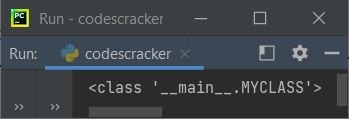As you can see from the above output, MYCLASS refers to the name of the class that object ob belongs to, where __main__ was the name of the module.

For example, to create an object named obj of the class MYCLASS, here is the code:

`obj = MYCLASS()`

### Accessing Attributes of the Class

To access an attribute of a class in Python, here is the syntax:

`object_name.variable_of_class`

For example, to access attributes of the class MYCLASS, created above ,here is the code:

```class MYCLASS:
num = 10
name = "Python"

obj = MYCLASS()
print(obj.num)
print(obj.name)```

The above Python program produces the output as shown in the snapshot given below: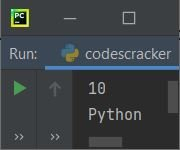That is, you can access attributes of the class just by using the dot (.) operator after the object. Class methods can be accessed by using the class name. One class can have multiple objects. Different objects have different behaviors. So we need to pass the object. The syntax is:

`class_name.method_name(object_name)`

For example:

```class MYCLASS:
def myfun(self):
print("I'm inside the method \"myfun()\".")
print("The method is inside the class \"MYCLASS\"")

ob = MYCLASS()
MYCLASS.myfun(ob)```

The output produced by the above program on a class and object in Python is: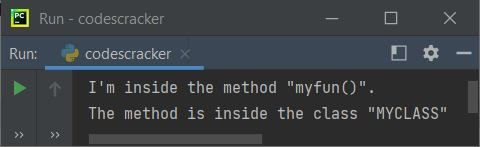Here, ob is passed to the myfun() method of the class MYCLASS. That is, the object is passed to "MYCLASS.myfun()," which is referred to as a parameter. As a result, this parameter (ob, the object) referred to the self (myfun() method parameter).

Another way to access the method of a class using its object is:

`object_name.method_name()`

For example:

```class MYCLASS:
def myfun(self):
print("I'm inside the method \"myfun()\".")
print("The method is inside the class \"MYCLASS\"")

ob = MYCLASS()
ob.myfun()```

This program produces exactly the same output as the previous program's output. From the above program, the following statement:

`ob.myfun()`

states that the object ob gets passed as a parameter to myfun(), as the object (ob) is of the class MYCLASS. Therefore, the myfun() method of this class gets accessed. This is essentially a Python shortcut for accessing the class's method. However, I do not recommend this method for beginners. I recommend using the previous way to access the method of a class using its object for all beginner learners.

## The __init__() method of the class

In Python, the double underscore before and after the variable and method referred to special variables and methods. So "__name__" is a special variable, whereas "__fun__()" is a special method.

The "__init__()" method is similar to the constructor. That is, upon creating an object of the class, it automatically calls this method once. For example:

```class MYCLASS:
def __init__(self):
print("I'm inside the method \"myfun()\".")
print("The method is inside the class \"MYCLASS\"")

ob = MYCLASS()```

produces the same output as the previous program. See, I've not called the method __init__(). I only created an object of the type MYCLASS. And this function automatically gets called after creating the object.

We can pass any number of arguments or parameters after "self" (self referring to the object itself). For example:

```class MYCLASS:
def __init__(self, name, rollno):
self.name = name
self.rollno = rollno

def myfun(self):
print("Name =", self.name)
print("Roll Number =", self.rollno)

ob = MYCLASS("John Harvard", 468)
MYCLASS.myfun((ob))```

Here is its sample output: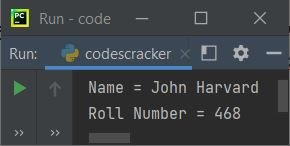In the above program, inside the class, "self" refers to an object. So, using the following statements:

```self.name = name
self.rollno = rollno```

initializes the value of name to a variable of the current object. In the same way, in method "myfun()," since "name" and "rollno" are not local variables, and since these two variables belong to object, "self" refers to the current object. So we need to use variables with "self." For example, the following example uses two objects:

```class MYCLASS:
def __init__(self, name, rollno):
self.name = name
self.rollno = rollno

def myfun(self):
print("Name =", self.name)
print("Roll Number =", self.rollno)

ob1 = MYCLASS("John Harvard", 468)
MYCLASS.myfun((ob1))

ob2 = MYCLASS("Jane Stanford", 4704)
MYCLASS.myfun((ob2))```

This program produces: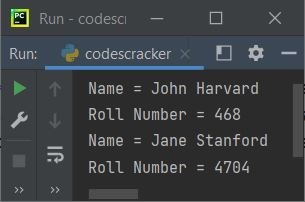The above program can also be created as:

```class MYCLASS:
def __init__(self, name, rollno):
self.name = name
self.rollno = rollno

ob1 = MYCLASS("John Harvard", 468)
print("Name =", ob1.name)
print("Roll Number =", ob1.rollno)

ob2 = MYCLASS("Jane Stanford", 4704)
print("Name =", ob2.name)
print("Roll Number =", ob2.rollno)```

That is, to create an instance of a class in Python, call the class using the class name and then pass the number of arguments that the __init__() method accepts. Here is an example.

```stud1 = STUDENT("Matt Damon", 198349)
stud2 = STUDENT("John F. Kennedy", 213590)
stud3 = STUDENT("Al Gore", 189392)```

Note: The method _init_() is a special method called the "class constructor" or "initialization method" that Python calls when you create a new instance of the class. You are free to declare other class methods just like normal functions in Python.

### The "self" parameter of a class's method

The "self" is basically referenced to the current object, or instance, of a class. It is used to access variables that belong to the class.

Important: There is no need to use only "self" to reference the current instance of the class. We can use any variable depending on our need, mood, or whatever you want to call it. But the condition is that this must be available as the first parameter of the method inside the class. For example:

```class MYCLASS:
def __init__(cc, name, rollno):
cc.name = name
cc.rollno = rollno

ob1 = MYCLASS("John Harvard", 468)
print("Name =", ob1.name)
print("Roll Number =", ob1.rollno)```

produces:

```Name = John Harvard
Roll Number = 468```

Note: But it is a better practice to use "self" to avoid any misunderstanding for yourself or for others who see your code.

## Delete an object in Python

To delete an object in Python, we need the "del" keyword. Here is the syntax to delete an object in Python:

`del object_name`

For example:

```class MYCLASS:
def __init__(cc, name, rollno):
cc.name = name
cc.rollno = rollno

ob1 = MYCLASS("John Harvard", 468)

del ob1

print("Name =", ob1.name)
print("Roll Number =", ob1.rollno)```

produces: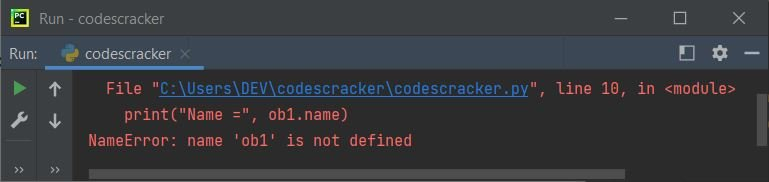Since the object is deleted immediately after creation. Therefore, accessing anything of the class using the object produces an error, as shown in the snapshot given above.

### The Application of the Pass Statement in Class

Since the class's definition cannot be empty. Therefore, if you need to create a class for further or future use, you do not need to define or provide any attributes, methods, or both for the class. Then you can use the pass statement or keyword to achieve this, so that the compiler does not produce any error messages while executing the code. For example:

```class class_name:
pass```

## Python Class and Object Example

Now, I think you have a complete understanding of classes and objects in Python. So let's finalize the thing with one last example given below:

```class STUDENT:
totalStudent = 0

def __init__(self, name, fee):
self.name = name
self.fee = fee
STUDENT.totalStudent += 1

def displayStudent(self):
print("Name:", self.name, end="")
print("\tFee:", self.fee)

stud1 = STUDENT("Matt Damon", 198349)
stud2 = STUDENT("John F. Kennedy", 213590)
stud3 = STUDENT("Al Gore", 189392)

stud1.displayStudent()
stud2.displayStudent()
stud3.displayStudent()

print("\nTotal Student = %d" % STUDENT.totalStudent)```

Here is its sample output: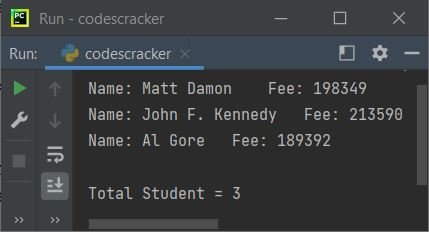Note: In the above program, the end parameter in print() is used to skip the insertion of an automatically generated newline.

The following statement appears in the preceding program:

`print("\nTotal Student = %d" % STUDENT.totalStudent)`

can also be moved inside any method of the class STUDENT. For example, the program given below produces the same output as the previous program:

```class STUDENT:
totalStudent = 0

def __init__(self, name, fee):
self.name = name
self.fee = fee
STUDENT.totalStudent += 1

def displayCount(self):
print("\nTotal Student = %d" % STUDENT.totalStudent)

def displayStudent(self):
print("Name:", self.name, end="")
print("\tFee:", self.fee)

stud1 = STUDENT("Matt Damon", 198349)
stud2 = STUDENT("John F. Kennedy", 213590)
stud3 = STUDENT("Al Gore", 189392)

stud1.displayStudent()
stud2.displayStudent()
stud3.displayStudent()

stud1.displayCount()```

You can call the displayCount() method with any of the three objects, say stud1, stud2, or stud3. Because the "__init__()" method is called automatically after the creation of each object of the same class, therefore, because I've created three objects, the method gets called three times. That increases the value of the variable "totalStudent" by 1 each time.

Here, the variable "totalStudent" is a class variable whose value is shared among all the instances of this class. This can be simply accessed as "STUDENT.totalStudent" from inside the class or from outside the class.

Python Online Test

« Previous Tutorial Next Tutorial »